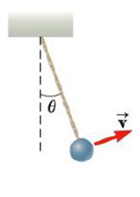Chapter 7, Problem 19P

Chapter
Section
Textbook Problem

One end of a cord is fixed and a small 0.500-kg object is attached to the other end, where it swings in a section of a vertical circle of radius 2.00 in, as shown in Figure P7.19. When 9 = 20.0°, the speed of the object is 8.00 m/s. At this instant, find (a) the tension in the string, (b) the tangential and radial components of acceleration, and (c) the total acceleration, (d) Is your answer changed if the object is swinging down toward its lowest point instead of swinging up? (e) Explain your answer to part (d).Figure P7.19

(a)

To determine
The tension in the string.

Explanation

Given Info: The mass of the object is 0.500kg , the radius of the circle that the object is swinging is 2.0m , the speed of the object is 8.0m/s and the angle of swing is 20.0°

Explanation:

When the object is swing to an angle 20.0° , the tension on the string will be the sum of the radial component of weight of the object and the centripetal force.

Express the relation for the tension in the string.

T=mgcosθ+mv2r

• θ is the angle of swing of object.
• m is the mass of object.
• g is acceleration due to gravity.
• v is the speed of the object.
• r is the radius of the circle that the object is swinging.

On re-arranging, the formula to calculate the tension of the string is,

T=m(v2r+gcosθ)

Substitute 0

(b)

To determine
The tangential and radial components of accelerations.

(c)

To determine
The total acceleration.

(d)

To determine
whether the answers will change if the object is swinging down towards its lowest point instead of swinging up.

(e)

To determine
The explanation for part (d).

Still sussing out bartleby?

Check out a sample textbook solution.

See a sample solution

The Solution to Your Study Problems

Bartleby provides explanations to thousands of textbook problems written by our experts, many with advanced degrees!

Get Started